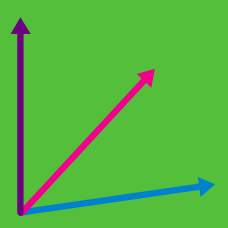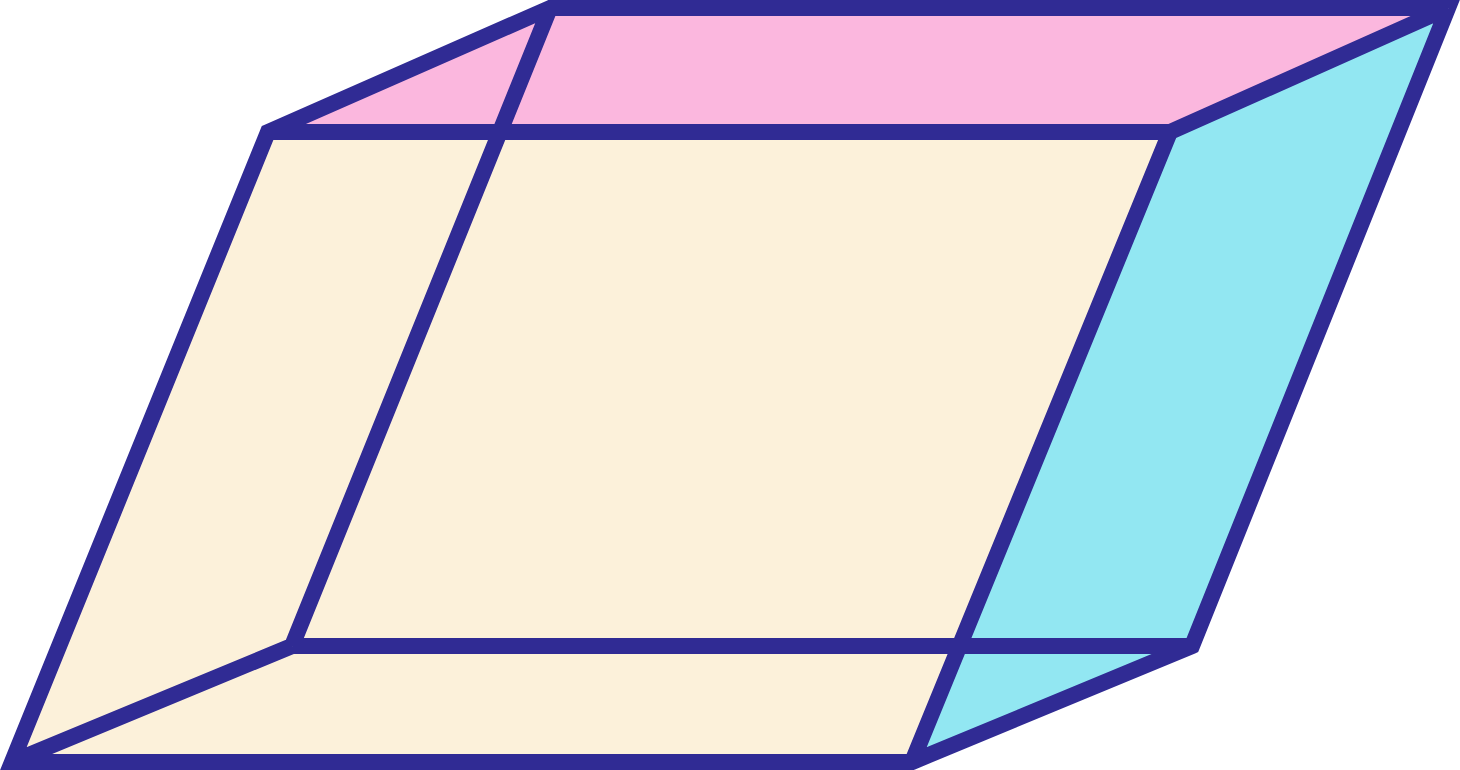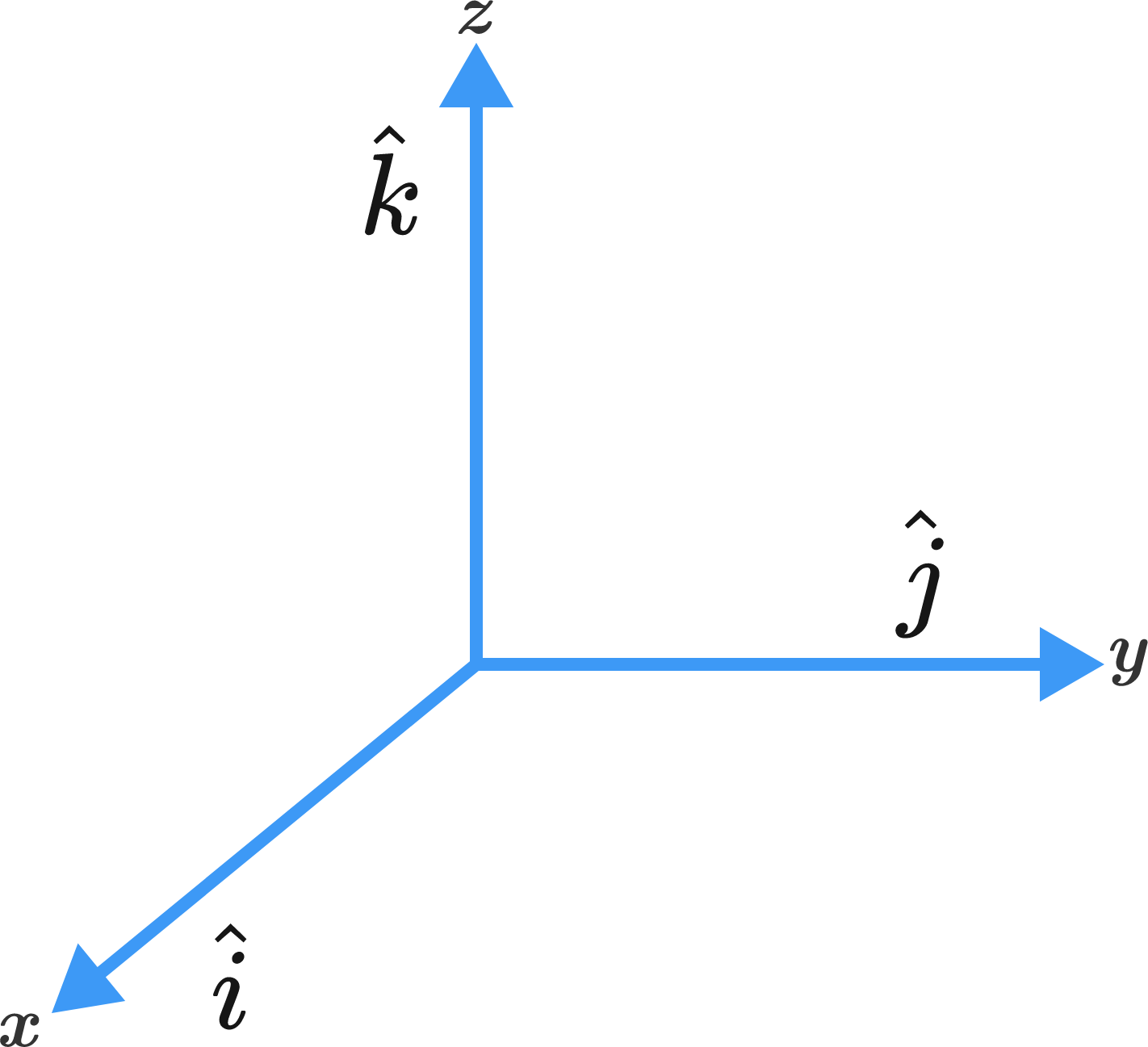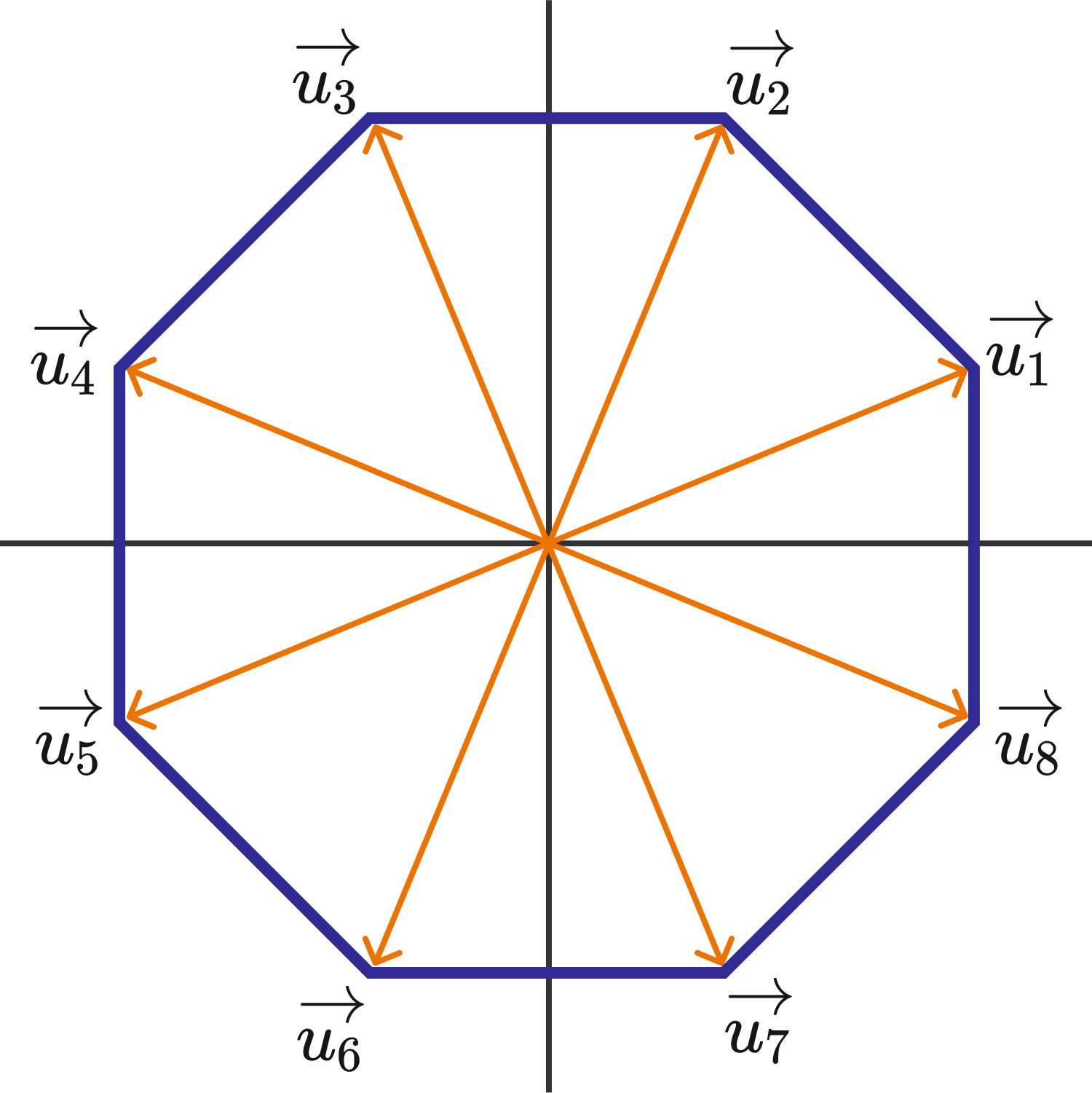Geometry

# Cross Product of Vectors: Level 3 Challenges

Consider the force vector $$\textbf{F} = \langle1, 1, \frac{1}{2}\rangle$$ . If the magnitude of the torque $$\tau = \overline{OP}\times\textbf{F}$$ is equal to the area of the equilateral triangle formed by the origin, $$P$$, and $$(1, -1, 1)$$, then determine the acute angle formed by $$\overline{OP}$$ and $$\textbf{F}$$. Give your answer in degrees.

Let $$\vec{a},\vec{b}$$ and $$\vec{c}$$ be three non-zero vectors such that no two of them are collinear and $$(\vec{a} \times \vec{b}) \times \vec{c}=\frac{1}{3} |\vec{b}||\vec{c}| \vec{a}$$. If $$\theta$$ is the angle between vectors $$\vec{b}$$ and $$\vec{c}$$, then the value of $$\sin\theta$$ is :Let $$\hat{u}$$ and $$\hat{v}$$ be unit vectors and $$\vec{w}$$ be a vector such that $$\vec{w}+(\vec{w}\ \times \hat{u})$$ $$=$$ $$\hat{v}$$.

The angle in degrees between $$\hat{u}$$ and $$\hat{v}$$ such that $$|(\hat{u} \times \hat{v}) \cdot \vec{w}|$$ is maximized is $$\theta$$ and the maximum value of $$|(\hat{u} \times \hat{v}) \cdot \vec{w}|$$ is $$M$$. Find the value of $$\theta + M$$.

$$Image$$ $$Credit :$$ $$Wikipedia$$Suppose $$\vec{p} , \vec{q}, \text{ and } \vec{r}$$ are three mutually perpendicular unit vectors.

Vector $$\vec{u}$$ satisfies the equation $\vec{p}\times((\vec{u} - \vec{q})\times\vec{p}) \hspace{.15cm} + \hspace{.15cm} \vec{q}\times((\vec{u} - \vec{r})\times\vec{q}) \hspace{.15cm} + \hspace{.15cm}\vec{r}\times((\vec{u} - \vec{p})\times\vec{r}) = 0$

What is $$\vec{u}$$ in terms of $$\vec{p} , \vec{q}, \text{ and } \vec{r}$$?Consider the regular octagon centered at the origin as shown at right. Eight unit vectors are drawn from the center of the octagon to each of its vertices and labeled in the figure. For each pair of distinct unit vectors $$\vec{u}_i, \vec{u}_j$$ with $$i < j$$, their cross product is computed.

What is the sum of all of these cross products?

×

Problem Loading...

Note Loading...

Set Loading...